Next: 3.2 Thermoelectrics of Graphene Antidot Lattices Up: 3.1 Thermoelectric Properties of AGNRs Previous: 3.1.1.3 Thermoelectric Figure of Merit   Contents

## 3.1.2 The Role of Line-Edge-Roughness

As shown in Sec. 3.1.1, the high lattice thermal conductance of AGNRs causes a small ballistic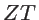value. In fact, A high thermal conductivity in the range of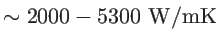has been reported for wide suspended single-layer graphene [28,72], which is only weakly affected by the boundary and substrate scatterings. On the other hand, in narrow AGNRs, line-edge-roughness (LER) is the dominant scattering source for both electron and phonon transport [73,74,70,75]. Therefore, in this section, we examine how LER scattering in very narrow AGNRs can affect the thermal and thermoelectric properties.Very recently, the effect of rough boundaries has been studied in defective AGNRs [76,77,70,78,79,80]. Those previous works have used a simple model for line-edge-roughness which neglect the correlation of the roughness. However, due to the wave nature of phonos, the correlation length of roughness plays an important role as well. To model LER-limited thermal conductivity, an exponential autocorrelation function is employed :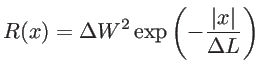(3.1)

the Fourier transform of which is the power spectrum of the roughness. In Eq. 3.1,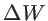is the root mean square of the roughness amplitude and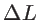is the roughness correlation length. The LER in the real space is achieved by adding a random phase to the power spectrum followed by an inverse Fourier transform [81,74]. Many samples with the same roughness parameters are generated and their thermal properties are evaluated by taking an ensemble average.

To investigate the effect of LER on the thermal conductivity and the MFP of phonons, we performed simulations on a statistical sample of 16-AGNR with roughness parameters of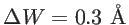and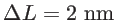. The main results are, however, almost independent of the actual value of the roughness parameters. The statistical average of the transmission function is shown in Fig. 3.7 for a channel length of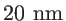. For reference, the ballistic transmission of a 16-AGNR is also shown with solid line.

Figure 3.7 compares the phonon transmission functions of a 16-AGNR assuming perfect edges and rough edges with roughness parameters ofand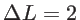nm. By introducing LER, the tranmission decreases from the ballistic value almost over entire energy range. To quantify the dependence of the transmission function on the channel length, the phonon MFP is defined as [32,57]: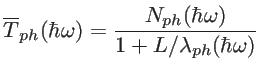(3.2)

where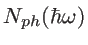is the ballistic transmission function and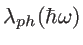is the phonon MFP at energy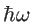.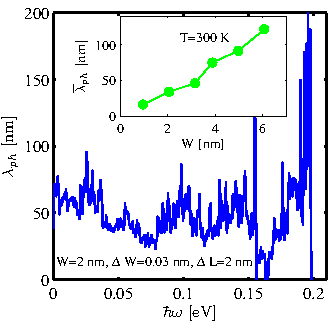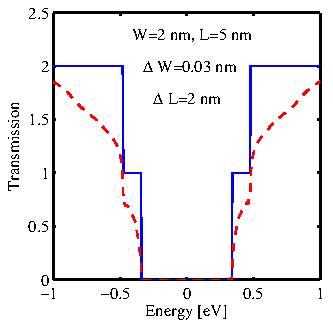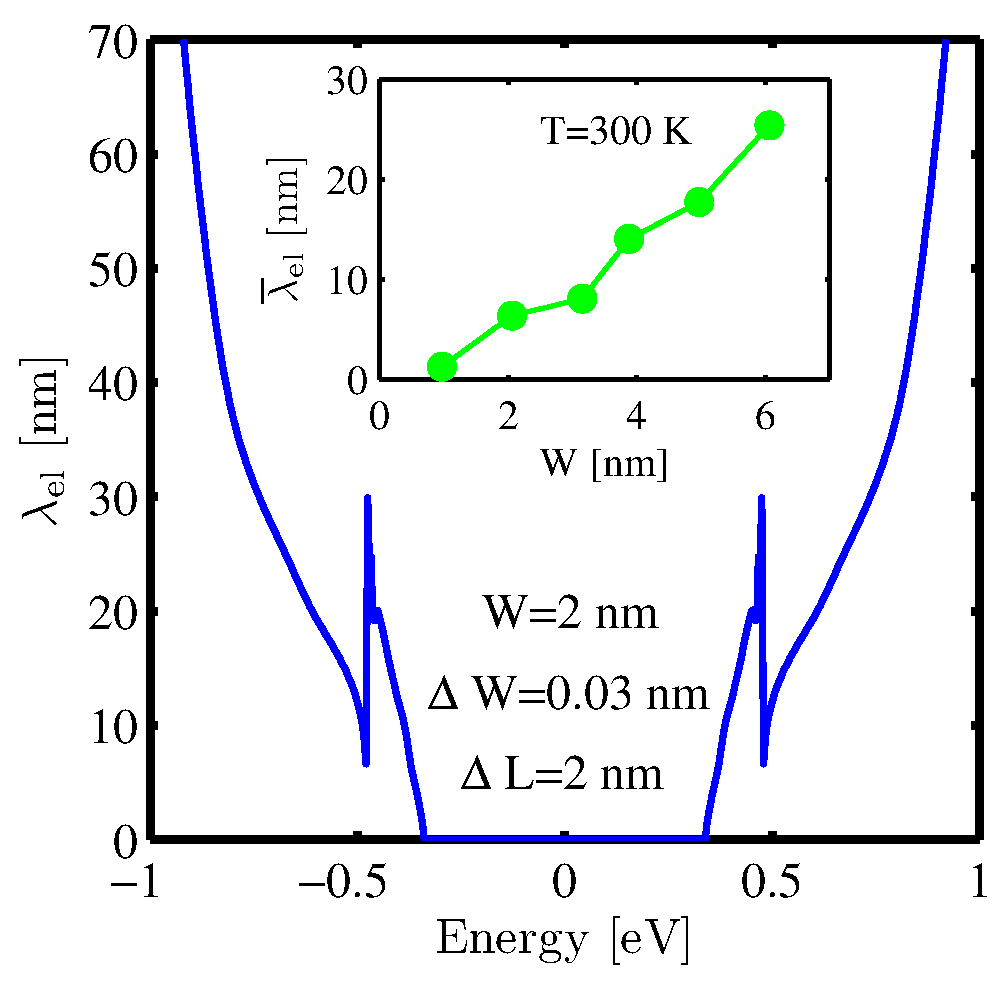Figure. 3.8 shows the phonon MFP of a 16-AGNR as a function of phonon frequency, which is around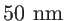. However, to study the dependence of the thermal conductance on the channel length one can define an effective MFP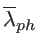as :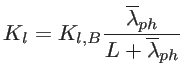(3.3)

which covers the contribution of phonons of different frequencies. Here, the ballistic thermal conductance of AGNRs with perfect edges is denoted by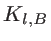. In the inset of Fig. 3.8 the effective room temperature MFP is shown for ribbon's of different width. The symbols in Fig. 3.8 are ensemble average values. As a rough estimate, the standard deviations of various quantities calculated in this section are about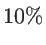of the corresponding average value for short and narrow channels, whereas they decrease to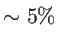of the average values for long and wide channels. By increasing the ribbon's width from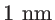to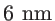the effective MFP increases linearly from aboutto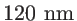. The phonon MFP of GNRs with smooth edges was reported to be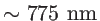. Therefore, it seems that LER degrades the high thermal conductivity of AGNRs and can help one achieves higher values of.

To examine the effect of LER on the electronic structure, the electronic transmission of a 16-AGNR with the channel length of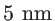is shown in Fig. 3.9, with and without the LER. LER degrades the transport of electrons at different energies. However, the reduction is lower at energies near the sub-band edges, where the density of states and thus the scattering rate is higher. Therefore, the presence of LER degrades the sharp features of electronic transmission function and the thermoelectric power factor . The corresponding MFP is shown in Fig. 3.10. The MFP is very small near the band edges due to the high density of states and thus high scattering rate. This indicates that the diffusive transmission function is very negligible near the first conduction subband (Fermi energy), which can drastically degrade the transport parameters. The effective MFP is also shown in the inset of Fig. 3.10. It increases almost nearly with the ribbon's width. However it is smaller than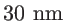even forAGNR. The small electron MFP can be described by considering the sensitivity of the band-gap to the ribbons width. The band-gap is not only inversely dependent on the ribbon's width, it is strongly dependent to the index of the ribbon either. Therefore, the presence of LER is not a small perturbation for the electron transport. In fact, the electron transmission will be in the localization regime and one should even define the localization length instead of mean-free-path for long channel . As the ratio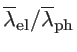is considerably smaller than one, we conclude that the presence of boundary scattering is not appropriate for the thermoelectric performance of AGNR-based devices, in contrast to what has been observed in silicon nanowires .

Finally, the inverse ofat room temperature as a function of ribbon's width and channel length is shown in Fig. 3.11. For very short channels, in which the transport is almost ballistic, the value ofis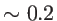for narrow ribbons and it decreases by increasing the width, as described in Sec. 3.1.1. However, the value ofdecreases by increasing the channel length, as the electron tranmission decreases faster than the phonon transmission function. For the channel length of about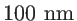,is less than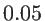, and even smaller values are expected for longer ribbons.In this section, the ballistic and diffusive thermoelectric properties of narrow AGNRs are comprehensively studied. The results indicate that the contribution of the second conduction subband increases the power factor. The second conduction is closer to the first one in wider ribbons. However, the small band-gap of wide AGNRs degrades the Seebeck coefficient. Designing an AGNR with large band-gap and closer conduction subbands is the way that could significantly improve the thermoelectric power factor. On the other hand, the high thermal conductance degrades the thermoelectric performance of AGNRs. Using line-edge-roughness for decreasing the thermal conductance degrades the thermoelectric properties, as it is more severe in the case of electron transport. Engineering AGNR-based materials in which the transport of phonons is more sensitive to the boundary scatting than that of electrons can help to improve or at leat retain thevalues in long ribbons. It might be accessible in some structures, in which the band-gap is not strongly related to the width. In the next couple of sections, these ideas are used in order to achieve high performance graphene-based thermoelectric devices.Next: 3.2 Thermoelectrics of Graphene Antidot Lattices Up: 3.1 Thermoelectric Properties of AGNRs Previous: 3.1.1.3 Thermoelectric Figure of Merit   Contents
H. Karamitaheri: Thermal and Thermoelectric Properties of Nanostructures